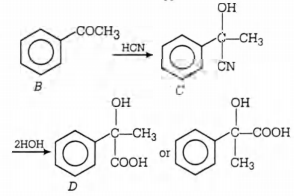# Q. In a set of reactions, acetic acid yielded a product D. $CH_3COOH\xrightarrow{SOCL_2} A\xrightarrow [anhy. AICI_3]{Benzene}B\xrightarrow {HCN}C\xrightarrow {HOH}D$ The structure of D would be

AIPMTAIPMT 2005Aldehydes Ketones and Carboxylic Acids

Solution:

## $CH_3COOH \xrightarrow {SOCI_2} \, \, _ { \, \, \, \, \, \, \, \, A}^{CH_3COCI} \xrightarrow [Anhy. AlCl_3] {Benzene}$You must select option to get answer and solution

## 1. An acidic buffer solution can be prepared by mixing the solution of

IIT JEE 1981 Equilibrium

## 2. A mixture of benzaldehyde and formaldehyde on heating with aqueous NaOH solution gives

IIT JEE 2001 Aldehydes Ketones and Carboxylic Acids

## 3. Which of the following solutions will have pH close to 1.0 ?

IIT JEE 1992 Equilibrium

## 4. Acetone is mixed with bleaching powder to give

AFMC 2004 Aldehydes Ketones and Carboxylic Acids

## 5. 75% of a first order reaction was completed in 32 minutes. When was 50% of the reaction completed

COMEDK 2010 Chemical Kinetics

## 6. The radii of $Na^+$ and $Cl^{-1}$ ions are 95 pm and 181 pm respectively. The edge length of NaCl unit cell is

KCET 2006 The Solid State